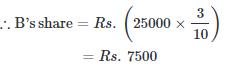# Test: Partnership 1

## 20 Questions MCQ Test Quantitative Techniques for CLAT | Test: Partnership 1

Description
This mock test of Test: Partnership 1 for Quant helps you for every Quant entrance exam. This contains 20 Multiple Choice Questions for Quant Test: Partnership 1 (mcq) to study with solutions a complete question bank. The solved questions answers in this Test: Partnership 1 quiz give you a good mix of easy questions and tough questions. Quant students definitely take this Test: Partnership 1 exercise for a better result in the exam. You can find other Test: Partnership 1 extra questions, long questions & short questions for Quant on EduRev as well by searching above.
QUESTION: 1

### Three partners shared the profit in a business in the ratio 5 : 7 : 8. They had partnered for 14 months, 8 months and 7 months respectively. What was the ratio of their investments?

Solution:

Let their investments be Rs. x for 14 months, Rs. y for 8 months and Rs. z for 7 months respectively.
Then, 14x : 8y : 7z = 5 : 7 : 8.
Now,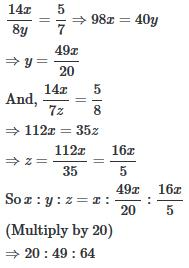QUESTION: 2

### Three partners A , B , C start a business . B's Capital is four times C's capital and twice A's capital is equal to thrice B's capital . If the total profit is Rs 16500 at the end of a year ,Find out B's share in it.

Solution:

Suppose C's capital = x then
B's capital = 4x (Since B's Capital is four times C's capital)
A's capital = 6x ( Since twice A's capital is equal to thrice B's capital)
A : B : C =6x : 4x : x
= 6 : 4 : 1
B's shareQUESTION: 3

### Kamal started a business investing Rs 9000. After five months, Sameer joined with a capital of Rs 8000. If at the end of the year, they earn a profit of Rs. 6970, then what will be the share of Sameer in the profit ?

Solution:

Now as per question, Kamal invested for 12 months and Sameer invested for 7 months.
So Kamal : Sameer = (9000 × 12) : (8000 × 7)
= 108 : 56
= 27 : 14
Sameer Ratio in profit will be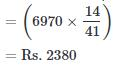QUESTION: 4

P and Q invested in a business. The profit earned was divided in the ratio 2 : 3. If P invested Rs 40000, the amount invested by Q is

Solution:

Let the amount invested by Q = q.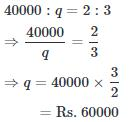QUESTION: 5

If 4 (P's Capital ) = 6 ( Q's Capital ) = 10 ( R's Capital ) , then out of the total profit of Rs 4650 , R will receive

Solution:

Let
P's capital = p,
Q's capital = q and
R's capital = r.
Then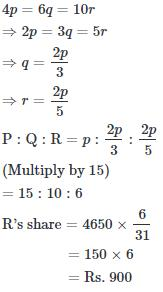QUESTION: 6

A, B, C rent a pasture. A puts 10 oxen for 7 months, B puts 12 oxen for 5 months and C puts 15 oxen for 3 months for grazing. If the rent of the pasture is Rs. 175, how much must C pay as his share of rent?

Solution:

A:B:C
=(10×7):(12×5):(15×3)
=70:60:45
=14:12:9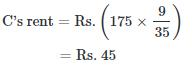QUESTION: 7

In a business, A and C invested amounts in the ratio 2 : 1 , whereas the ratio between amounts invested by A and B was 3 : 2 . If Rs 157300 was their profit, how much amount did B receive?

Solution:

Assume that investment of C = x
Then, investment of A = 2x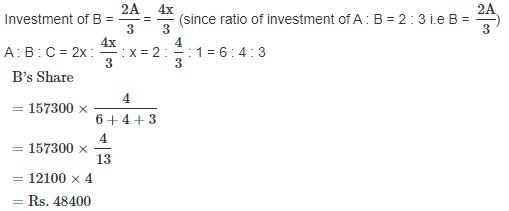QUESTION: 8

A and B started a business in partnership investing Rs. 20,000 and Rs. 15,000 respectively. After six months, C joined them with Rs. 20,000. What will be B's share in total profit of Rs. 25,000 earned at the end of 2 years from the starting of the business?

Solution:

A : B : C
= (20,000 × 24) : (15,000 × 24) : (20,000 × 18)
= 4 : 3 : 3.
So B's Share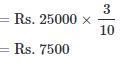QUESTION: 9

P, Q, R enter into a partnership. P initially invests 25 lakh & adds another 10 lakhs after one year. Q initially invests 35 lakh & withdrawal 10 lakh after 2 years and R invests Rs 30 Lakhs . In what ratio should the profit be divided at the end of 3 years?

Solution:

P : Q : R
= (25 × 1 + 35 × 2) : (35 × 2 : 25 × 1) : (30 × 3)
= 95 : 95 : 90
= 19 : 19: 18

QUESTION: 10

A, B, C subscribe Rs. 50,000 for a business. A subscribes Rs. 4000 more than B and B Rs. 5000 more than C. Out of a total profit of Rs. 35,000, A receives

Solution:

Let C = x.
Then, B = x + 5000 and A = x + 5000 + 4000 = x + 9000
So, x + x + 5000 + x + 9000 = 50000
⇒ 3x = 36000
⇒ x = 12000
A : B : C = 21000 : 17000 : 12000 = 21 : 17 : 12
So A's Share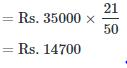QUESTION: 11

A and B invest in a business in the ratio 3 : 2. If 5% of the total profit goes to charity and A's share is Rs. 855, the total profit is:

Solution:

Let the total profitbe Rs.100 After paying to charity, A's share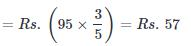If A's share is Rs.57,
Total profit=Rs.100
If A's share Rs.855,
Total profit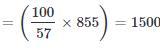QUESTION: 12

A, B and C jointly thought of engaging themselves in a business venture. It was agreed that A would invest Rs. 6500 for 6 months, B, Rs. 8400 for 5 months and C, Rs. 10,000 for 3 months. A wants to be the working member for which, he was to receive 5% of the profits. The profit earned was Rs. 7400. Calculate the share of B in the profit.

Solution:

For managing, A received = 5% of Rs. 7400 = Rs. 370.
Balance = Rs. (7400 - 370) = Rs. 7030.
Ratio of their investments = (6500 x 6) : (8400 x 5) : (10000 x 3)
= 39000 : 42000 : 30000
= 13 : 14 : 10
∴ B's Share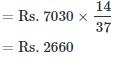QUESTION: 13

A, B and C enter into a partnership in the ratio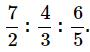After 4 months, A increases his share 50%. If the total profit at the end of one year be Rs. 21,600, then B's share in the profit is:

Solution:

Ratio of initial investments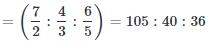Let the initial investments be
105x,40x and 36x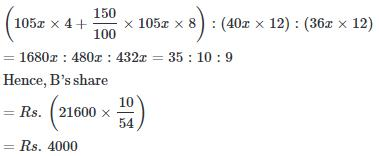QUESTION: 14

A, B, C subscribe Rs. 50,000 for a business. A subscribes Rs. 4000 more than B and B Rs. 5000 more than C. Out of a total profit of Rs. 35,000, A receives:

Solution:

Let C=x
Then, B=x+5000 and
A=x+5000+4000
=x+9000
So, x+x+5000+x+9000=50000
⇒3x=36000
⇒x=12000
A:B:C=21000:17000:12000
=21:17:12
∴A's share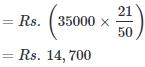QUESTION: 15

Three partners shared the profit in a business in the ratio 5 : 7 : 8. They had partnered for 14 months, 8 months and 7 months respectively. What was the ratio of their investments?

Solution:

Let their investments be
Rs.x for 14months,
Rs.y for 8 months and
Rs.z for 7 months respectively. Then,14x:8y:7z=5:7:8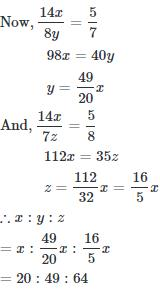QUESTION: 16

A starts business with Rs. 3500 and after 5 months, B joins with A as his partner. After a year, the profit is divided in the ratio 2 : 3. What is B's contribution in the capital?

Solution:

Let B's capital be Rs. x
∴ A's share in 12 months=3500 × 12
And, B's share in 7 months=7x
Let B's capitalbe Rs.x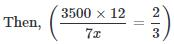⇒14x=126000
⇒x=9000

QUESTION: 17

A and B entered into partnership with capitals in the ratio 4 : 5. After 3 months, A withdrew 1/4 of his capital and B withdrew 1/5 of his capital. The gain at the end of 10 months was Rs. 760. A's share in this profit is:

Solution:

A:B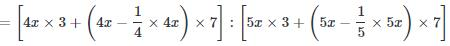=(12x+21x):(15x+28x)
=33x:43x
=33:43
∴A's share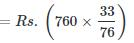=Rs.330

QUESTION: 18

A and B started a partnership business investing some amount in the ratio of 3 : 5. C joined then after six months with an amount equal to that of B. In what proportion should the profit at the end of one year be distributed among A, B and C?

Solution:

Let initial investment of A is 3x and B is 5x, then C investment is also 5x, but most important to note in this question is the time duration of investment
Like, A invested for 12 months, B invested for 12 months and C invested for 6 months.
A : B : C = (3x x 12) : (5x x 12) : (5x x 6)
= 36 : 60 : 30
= 6 : 10 : 5.

QUESTION: 19

A, B, C rent a pasture. A puts 10 oxen for 7 months, B puts 12 oxen for 5 months and C puts 15 oxen for 3 months for grazing. If the rent of the pasture is Rs. 175, how much must C pay as his share of rent?

Solution:

A:B:C
=(10×7):(12×5):(15×3)
=70:60:45
=14:12:9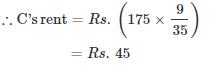QUESTION: 20

A and B started a business in partnership investing Rs. 20,000 and Rs. 15,000 respectively. After six months, C joined them with Rs. 20,000. What will be B's share in total profit of Rs. 25,000 earned at the end of 2 years from the starting of the business?

Solution:

A:B:C
=(20000×24):(15000×24):(20000×18)
=4:3:3